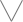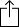C
D
E
F
G
A
B
Chord
Scale
Custom
3nps
CAGED
None
Notes
Degrees
Intervals
None

1
2
3
4
5
6
7
1
2
3
4
5
6
7
8
9
10
11
12
13
14
15
16
17
18
19
20
21
22
23
24
E
B
G
D
A
E
B
F
F
F
G
A
A
B
B
C
C
D
D
E
F
F
G
A
A
B
B
C
C
D
D
E
C
C
D
D
E
F
F
G
A
A
B
B
C
C
D
D
E
F
F
G
A
A
B
B
A
A
B
B
C
C
D
D
E
F
F
G
A
A
B
B
C
C
D
D
E
F
F
G
D
E
F
F
G
A
A
B
B
C
C
D
D
E
F
F
G
A
A
B
B
C
C
D
B
B
C
C
D
D
E
F
F
G
A
A
B
B
C
C
D
D
E
F
F
G
A
A
F
F
G
A
A
B
B
C
C
D
D
E
F
F
G
A
A
B
B
C
C
D
D
E
C
C
D
D
E
F
F
G
A
A
B
B
C
C
D
D
E
F
F
G
A
A
B
B
G
A
A
B
B
C
C
D
D
E
F
F
G
A
A
B
B
C
C
D
D
E
F
F

B Major Dominant Eleventh for 8 String Guitar - 3nps Chart

A B Major dominant eleventh chord, also written as "B11" or "Bdom11" contains the notes B, D#, F#, A, C# and E. The chord is comprised of the intervals M3, m3, m3, M3 and m3.

B
D
F
A
C
EM3
m3
m3
M3
m3
Play scaleand then